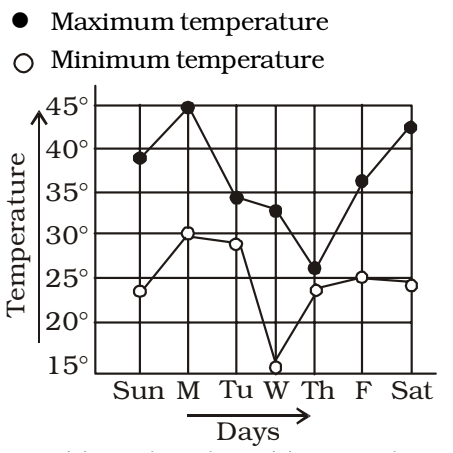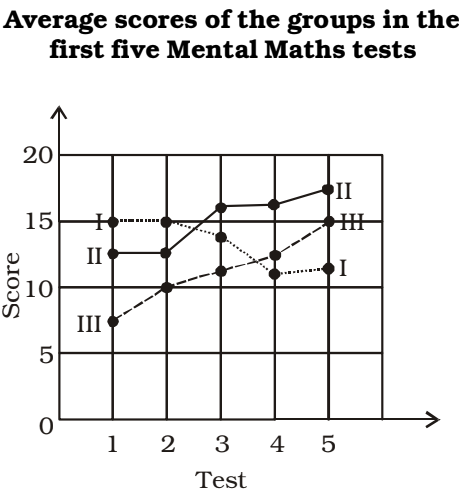## Introduction to Data Interpretation

#### Data Interpretation

Direction: Study the following graph and answer the questions.1. Find the percentage decrease in income from 2001 to 2002.

1. Definite income is not known.

##### Correct Option: D

Definite income is not known.
Hence , Data is not sufficient for solve this question .

Direction: The graph given below shows the daily income of 50 workers in a factory. Study the graph and answer the questions.1. The median wages in the factory is

1. According to given graph in question ,
Lowest value in wages = 120
Highest value in wages = 200

 Median = Lowest value + Highest value 2

##### Correct Option: D

According to given graph in question ,
Lowest value in wages = 120
Highest value in wages = 200

 Median = Lowest value + Highest value 2

 Median = 120 + 200 = \$ 160 2

1. What percentage of the factory workers earn between \$ 150 and \$ 180?

1. As per the given graph in question ,

Wages Number of workers
120 12
14026 - 12 = 14
160 34 – 26 = 8
180 40 – 34 = 6
200 50 – 40 = 10

From above given table , Number of workers earn between \$ 150 and \$ 180 = 8
Total number of workers = 12 + 14 + 8 + 6 + 10 = 50

##### Correct Option: B

As per the given graph in question ,

Wages Number of workers
120 12
14026 - 12 = 14
160 34 – 26 = 8
180 40 – 34 = 6
200 50 – 40 = 10

From above given table , Number of workers earn between \$ 150 and \$ 180 = 8
Total number of workers = 12 + 14 + 8 + 6 + 10 = 50
 ∴ Required percent = 8 × 100 = 16% 50

1. The following graph represents the maximum and minimum temperature recorded every day in a certain week. The day on which the difference between the maximum and minimum temperature was maximum is1. As per the given graph in question ,
Difference between temperatures :
Sunday ⇒ 39 – 23 = 16°
Saturday ⇒ 42.5 – 24 = 18.5°
Wednesday ⇒ 32.5 – 15 = 17.5°

##### Correct Option: B

As per the given graph in question ,
Difference between temperatures :
Sunday ⇒ 39 – 23 = 16°
Saturday ⇒ 42.5 – 24 = 18.5°
Wednesday ⇒ 32.5 – 15 = 17.5°
Hence , required answer is Saturday .

1. A class is divided into 3 equal groups and the class is given 5 tests in Maths. Average score of the groups and the tests is given below. The average score of the entire class in Test II is1. According to given graph in question ,
Sum of marks = 10 + 12.5 + 15
Total number of groups = 3

 Average marks = 10 + 12.5 + 15 3

##### Correct Option: D

According to given graph in question ,
Sum of marks = 10 + 12.5 + 15
Total number of groups = 3

 Average marks = 10 + 12.5 + 15 3

 Average marks = 37.5 = 12.5 3